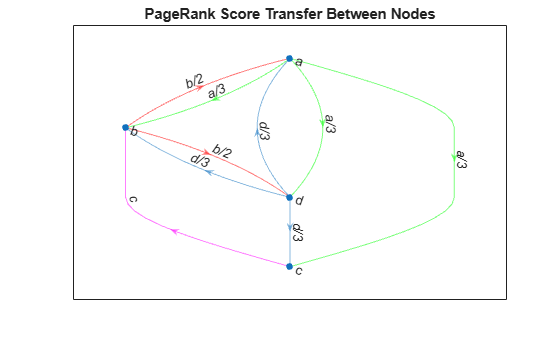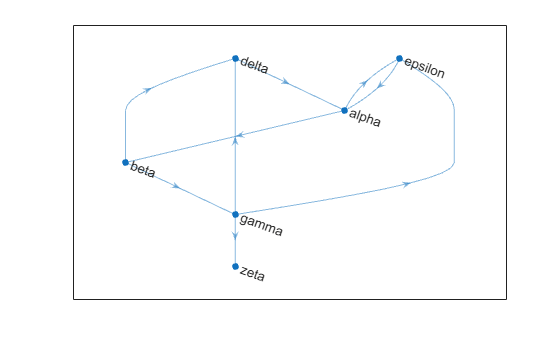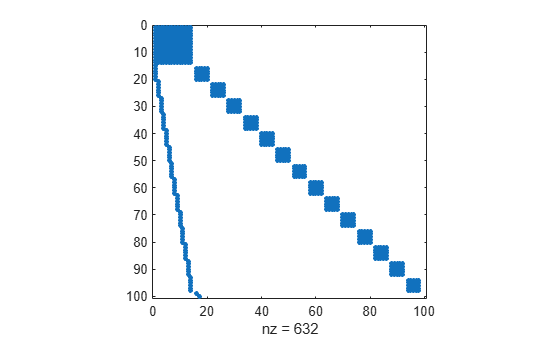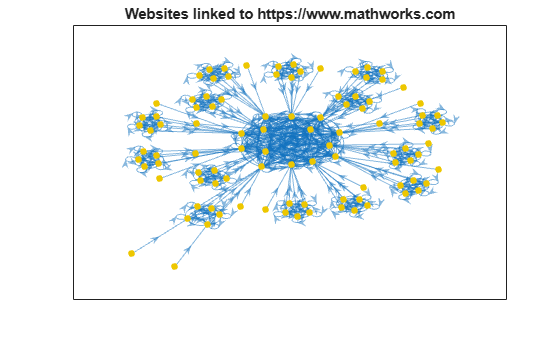# 使用 PageRank 算法对网站进行排名

### 算法说明

`r = (1-P)/n + P*(A'*(r./d) + s/n);`

• `r` 是 PageRank 得分的向量。

• `P` 是标量阻尼因子（通常为 0.85），这是随机浏览者点击当前网页上的链接而不是在另一随机网页上继续点击的概率。

• `A'` 是图形的邻接矩阵的转置。

• `d` 是包含图形中每个节点的出度的向量。对于没有外向边的节点，`d` 设置为 `1`

• `n` 是图形中节点的标量数量。

• `s` 是无链接的网页的 PageRank 得分的标量总和。

```s = {'a' 'a' 'a' 'b' 'b' 'c' 'd' 'd' 'd'}; t = {'b' 'c' 'd' 'd' 'a' 'b' 'c' 'a' 'b'}; G = digraph(s,t); labels = {'a/3' 'a/3' 'a/3' 'b/2' 'b/2' 'c' 'd/3' 'd/3' 'd/3'}; p = plot(G,'Layout','layered','EdgeLabel',labels); highlight(p,[1 1 1],[2 3 4],'EdgeColor','g') highlight(p,[2 2],[1 4],'EdgeColor','r') highlight(p,3,2,'EdgeColor','m') title('PageRank Score Transfer Between Nodes')````centrality` 函数包含用于计算 PageRank 得分的选项。

### 包含 6 个节点的 PageRank

```s = [1 1 2 2 3 3 3 4 5]; t = [2 5 3 4 4 5 6 1 1]; names = {'http://www.example.com/alpha', 'http://www.example.com/beta', ... 'http://www.example.com/gamma', 'http://www.example.com/delta', ... 'http://www.example.com/epsilon', 'http://www.example.com/zeta'}; G = digraph(s,t,[],names)```
```G = digraph with properties: Edges: [9x1 table] Nodes: [6x1 table] ```
```plot(G,'Layout','layered', ... 'NodeLabel',{'alpha','beta','gamma','delta','epsilon','zeta'})````pr = centrality(G,'pagerank','FollowProbability',0.85)`
```pr = 6×1 0.3210 0.1706 0.1066 0.1368 0.2008 0.0643 ```

```G.Nodes.PageRank = pr; G.Nodes.InDegree = indegree(G); G.Nodes.OutDegree = outdegree(G); G.Nodes```
```ans=6×4 table Name PageRank InDegree OutDegree __________________________________ ________ ________ _________ {'http://www.example.com/alpha' } 0.32098 2 2 {'http://www.example.com/beta' } 0.17057 1 2 {'http://www.example.com/gamma' } 0.10657 1 3 {'http://www.example.com/delta' } 0.13678 2 1 {'http://www.example.com/epsilon'} 0.20078 2 1 {'http://www.example.com/zeta' } 0.06432 1 0 ```

### mathworks.com 网站的 PageRank 得分

```load mathworks100.mat spy(A)````G = digraph(A,U)`
```G = digraph with properties: Edges: [632x1 table] Nodes: [100x1 table] ```

```plot(G,'NodeLabel',{},'NodeColor',[0.93 0.78 0],'Layout','force'); title('Websites linked to https://www.mathworks.com')``````pr = centrality(G,'pagerank','MaxIterations',200,'FollowProbability',0.85); G.Nodes.PageRank = pr; G.Nodes.InDegree = indegree(G); G.Nodes.OutDegree = outdegree(G);```

`G.Nodes(1:25,:)`
```ans=25×4 table Name PageRank InDegree OutDegree ______________________________________________________________________________ ________ ________ _________ {'https://www.mathworks.com' } 0.044342 20 14 {'https://ch.mathworks.com' } 0.043085 20 14 {'https://cn.mathworks.com' } 0.043085 20 14 {'https://jp.mathworks.com' } 0.043085 20 14 {'https://kr.mathworks.com' } 0.043085 20 14 {'https://uk.mathworks.com' } 0.043085 20 14 {'https://au.mathworks.com' } 0.043085 20 14 {'https://de.mathworks.com' } 0.043085 20 14 {'https://es.mathworks.com' } 0.043085 20 14 {'https://fr.mathworks.com' } 0.043085 20 14 {'https://in.mathworks.com' } 0.043085 20 14 {'https://it.mathworks.com' } 0.043085 20 14 {'https://nl.mathworks.com' } 0.043085 20 14 {'https://se.mathworks.com' } 0.043085 20 14 {'https://www.mathworks.com/index.html%3Fnocookie%3Dtrue' } 0.0015 0 1 {'https://www.mathworks.com/company/aboutus/policies_statements/patents.html'} 0.007714 6 6 ⋮ ```

```H = subgraph(G,find(G.Nodes.PageRank > 0.005)); plot(H,'NodeLabel',{},'NodeCData',H.Nodes.PageRank,'Layout','force'); title('Websites linked to https://www.mathworks.com') colorbar```### 参考

Moler, C. Experiments with MATLAB.Chapter 7:Google PageRank.MathWorks, Inc., 2011.